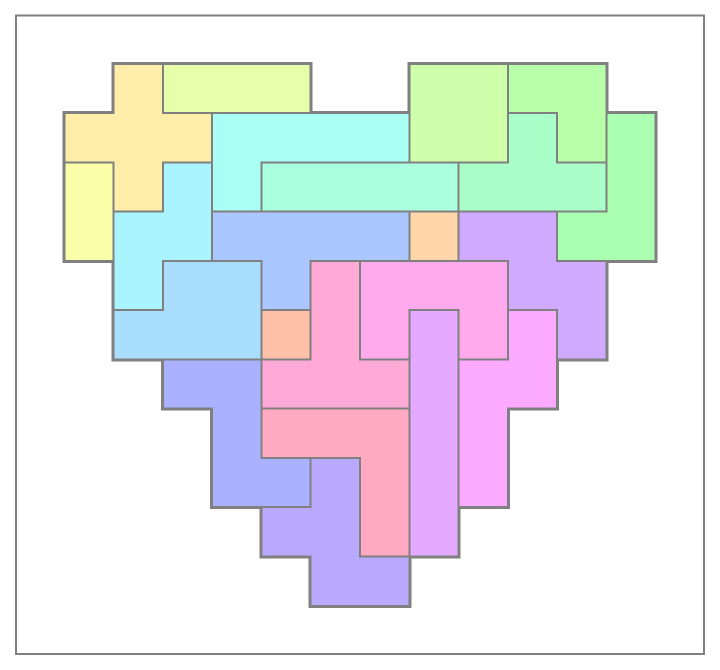Function Repository Resource:

# PolyominoPlot

Represent an array as polyomino regions

 ResourceFunction["PolyominoPlot"][mat] for matrix mat, aggregates adjacent identical values and plots the result as a configuration of polyominoes.

## Details

ResourceFunction["PolyominoPlot"] is similar to ArrayPlot but only draws edges between dissimilar values
The output of PolyominoPlot is a Graphics object.
PolyominoPlot accepts all the options of Graphics, as well as ColorRules and ColorFunction.
PolyominoPlot accepts an additional option "GraphicsStyle", which directly feeds style primitives into the first argument of the underlying Graphics.

## Examples

### Basic Examples (3)

Plot a chair tromino next to a monomino:

 In:=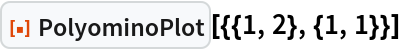Out=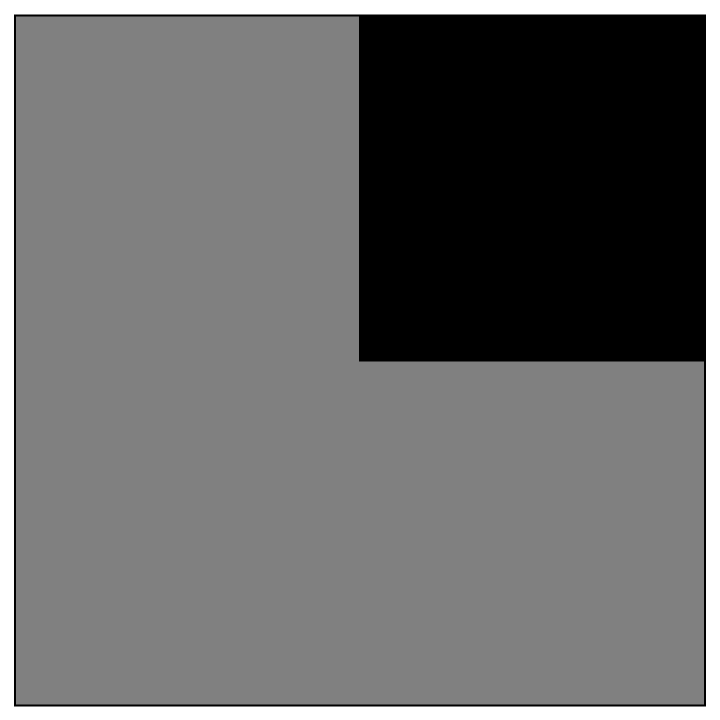Plot two chair trominoes next to three monominoes:

 In:=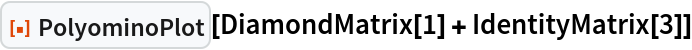Out=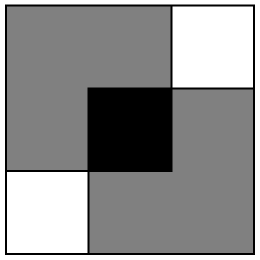A random polyomino plot over four values:

 In:=Out=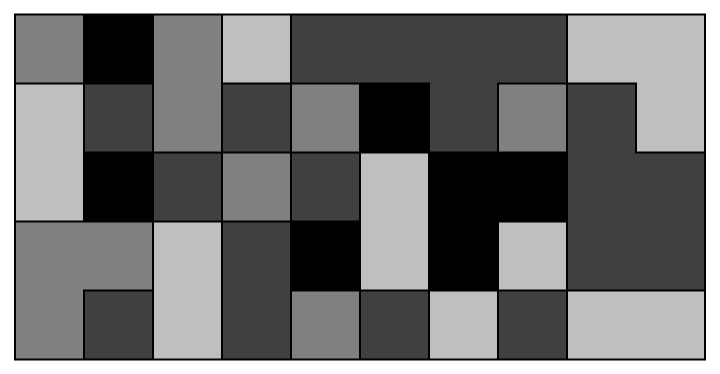### Options (2)

 In:=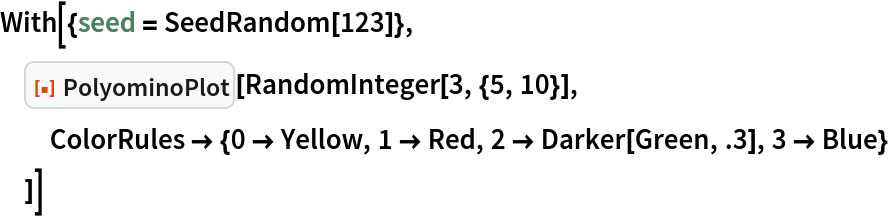Out=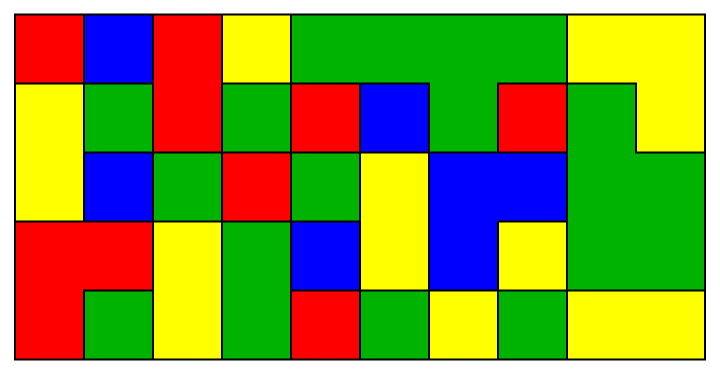Colors can also be added by setting ColorFunction:

 In:=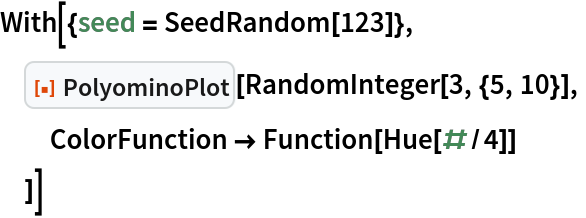Out=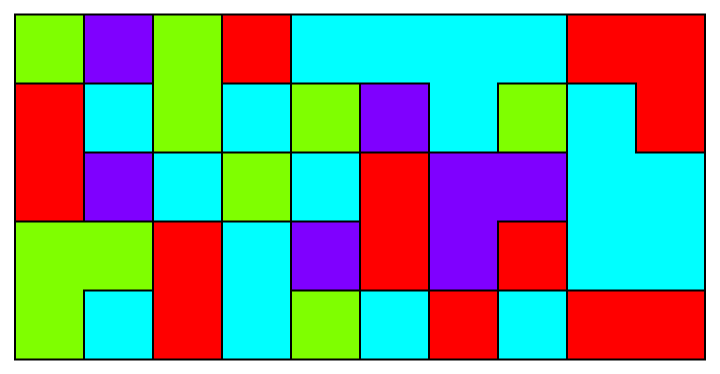Override the edge style interior to Graphics:

 In:=Out=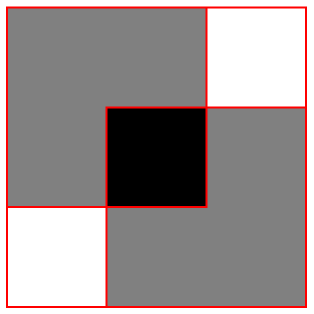### Properties and Relations (3)

PolyominoPlot differs from ArrayPlot in its ability to draw region boundaries:

 In:=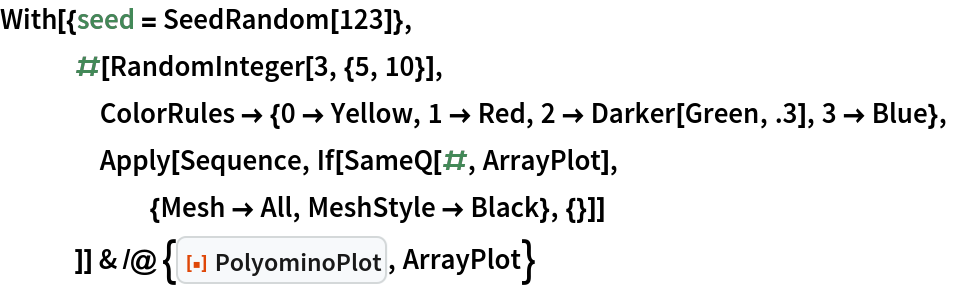Out=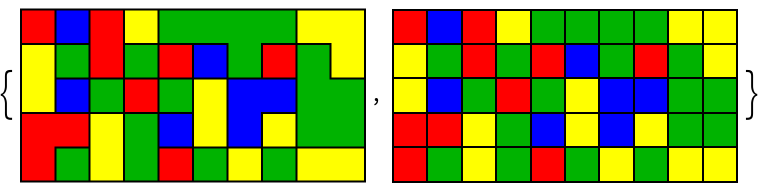Unlike ArrayPlot, colors are not strictly necessary:

 In:=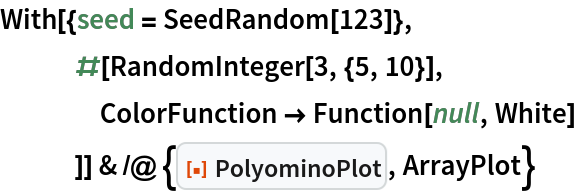Out=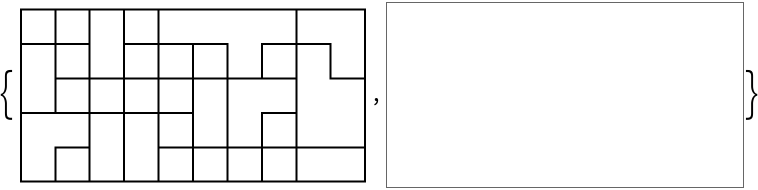PolyominoPlot can achieve finer results than ArrayMesh:

 In:=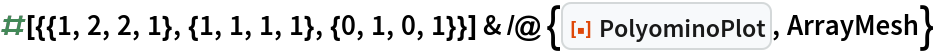Out=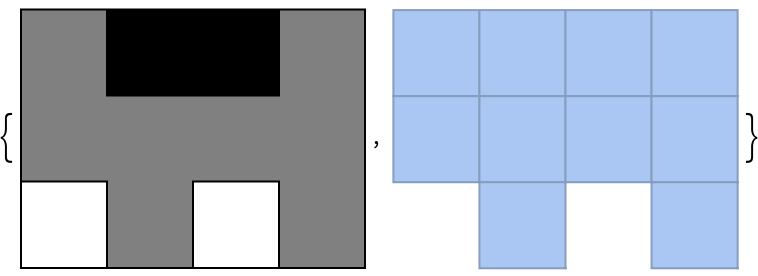### Neat Examples (2)

Plot a solution to Scott's pentomino problem:

 In:=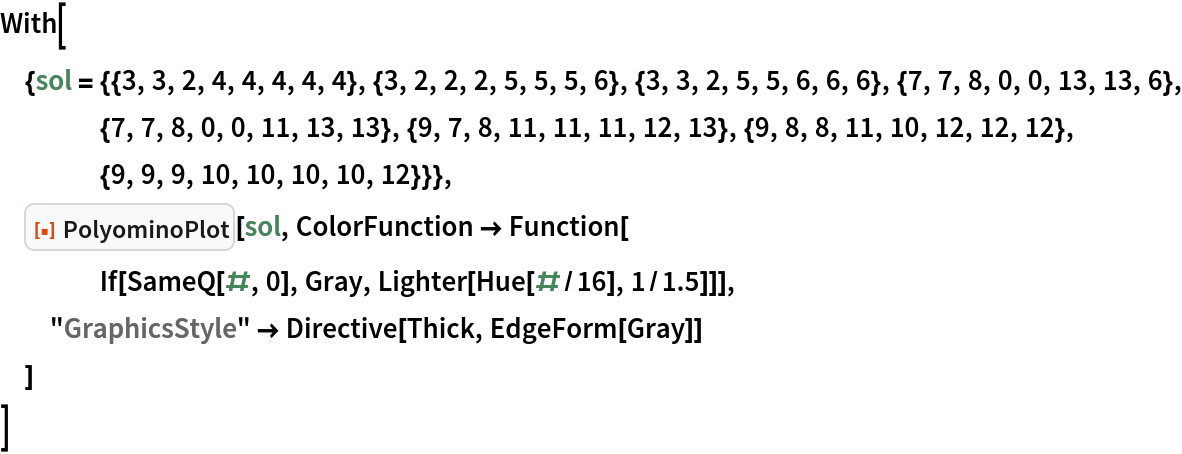Out=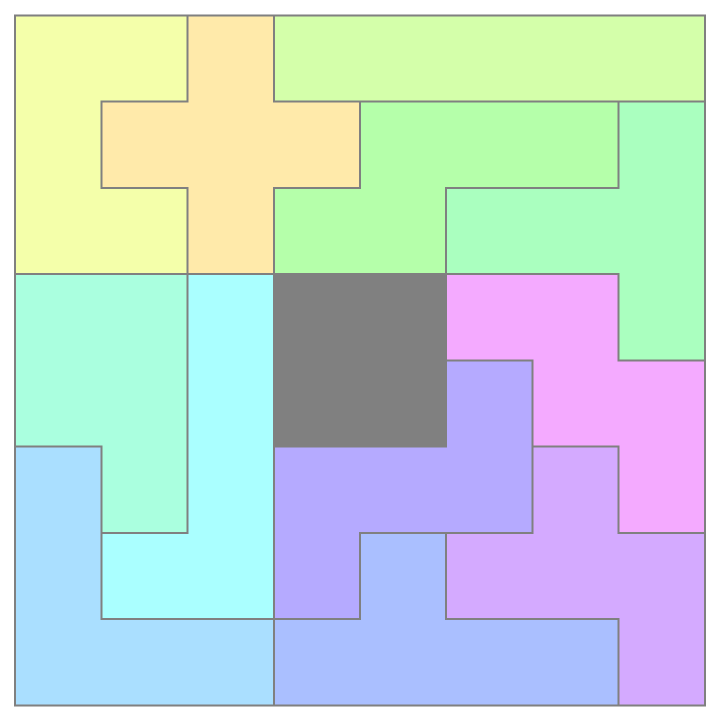Plot a solution to the heart puzzle:

 In:=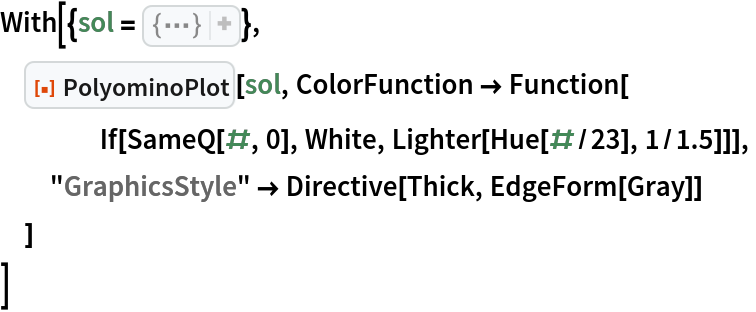Out=